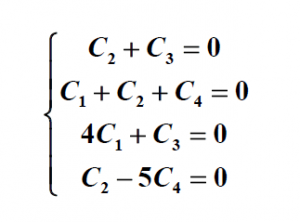# Check Out This Example of Polynomial With Solution

Check whether the polynomials p1(x)=x2+4x, p2(x)=1+x3+x2, p3(x)=x3+x, p4(x)=x2-5 form a basis in the space P3.

Solution:

The standard basis in the space P3 look like {1, x, x2, x3}, and they have 4 basis elements. To prove that polynomials p1, p2, p3, p4 form a basis in the space P3 it is sufficient to show that they are linearly independent.

Let’s form a linear combination:

C1 p1 ( x) + C2 p2 ( x) + C3 p3 ( x) + C4 p4 ( x) = C1  (x 2 +4 x) +  C2  (1 +  x 3 +x 2 ) + C3  (x 3 + x) + C4 ( x 25) = C1 x 2 + C1 4 x  + C2  + C2 x 3 + C2 x 2  + C3 x 3 + C3 x + C4 x 2  – C4 5 =

x 3 (C2 + C3 ) + x 2  (C1 + C2 + C4 ) +  x (4C1 +  C3   +  C25C4 )

This linear combination is equal to zero only if all coefficients are zero at powers of x, so we have come to the system:The system’s only solution is C1 = C2=C3 = C4 = 0 which means that the polynomials  p1, p2, p3, p4 are linearly independent and therefore form a basis in the space P3.

In this example of polynomial with solution, you can find the answer to the question that was set by our professor. We want to inform you that AssignmentShark.com can help you with your practical tasks. Since each student faces difficulties while studying from time to time, we have made it so that anyone can get help from our experts. There is a very successful solution to your problem with homework – place an order on our site. Tell us what your requirements are and don’t forget to set the deadline while placing the order.

If you are not much involved in math, our expert can solve any of your problems. Our service is used by numerous students and all of them remain satisfied. How many assignments do you need help with? On our site, you can get help with all of your tasks – just request do my homework online. Thus, you can spend your time as you want while our expert is dealing with your order. We can deal with different types of assignments. Can you become successful in your study? Of course – simply contact us right now!